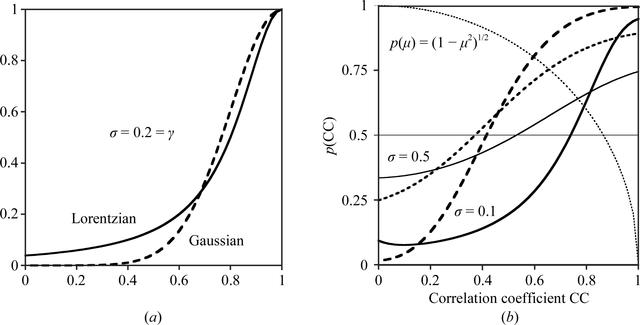disable zoom     view article Figure 6 Probability functions for correlation coefficients. (a) Comparison of Gaussian (dashed line) and Cauchy–Lorentzian (solid line) distributions with mean 1.0 and width parameter (σ or γ) = 0.2; the Lorentzian distribution has more extensive tails. (b) The effect on the modelled distribution p(CC) of σ(CC) and including p(μ) = (1 − μ2)1/2 (dotted line). A larger value of σ(CC) broadens the distribution (thin lines, σ = 0.5; thick lines, σ = 0.1). The effect of including the p(μ) term (solid lines) is to shift the point at which p(CC) rises above 0.5 to a larger value of CC than without it (dashed lines).BIOLOGICALCRYSTALLOGRAPHY
ISSN: 1399-0047
Volume 67| Part 4| March 2011| Pages 282-292